# How to merge multiple worksheets into one worksheet

This post explains that how to combine multiple excel worksheets into one without copying and pasting. How to merge two or more excel worksheet into one worksheet by using some VBA code. How to consolidate data from multiple worksheets into one worksheet in excel.

## Merge multiple worksheets into one worksheet

You may often need to merge multiple worksheets into one worksheet, which makes it easier for us to quickly count and analyze the data. If you just merge few worksheets, then you can just only use copying or pasting method, but if there are lots of worksheets, and this is not a good method to do it. At this time, you can write a new excel macro to merge multiple worksheets in excel VBA.

You can create a new excel macro to combine multiple worksheets into one worksheet in Excel VBA, just refer to the below steps:

1# click on “Visual Basic” command under DEVELOPER Tab.2# then the “Visual Basic Editor” window will appear.

3# click “Insert” ->”Module” to create a new module4# paste the below VBA code into the code window. Then clicking “Save” button.

```Sub MergeMultipleWorksheet()
Dim C As Integer
On Error Resume Next
Sheets(1).Select
Sheets(1).Name = "MergedSheet"
Sheets(2).Activate
Range("A1").EntireRow.Select
Selection.Copy Destination:=Sheets(1).Range("A1")
For C = 2 To Sheets.Count
Sheets(C).Activate
Range("A1").Select
Selection.CurrentRegion.Select
Selection.Offset(1, 0).Resize(Selection.Rows.Count - 1).Select
Selection.Copy Destination:=Sheets(1).Range("A65536").End(xlUp)(2)
Next
End Sub```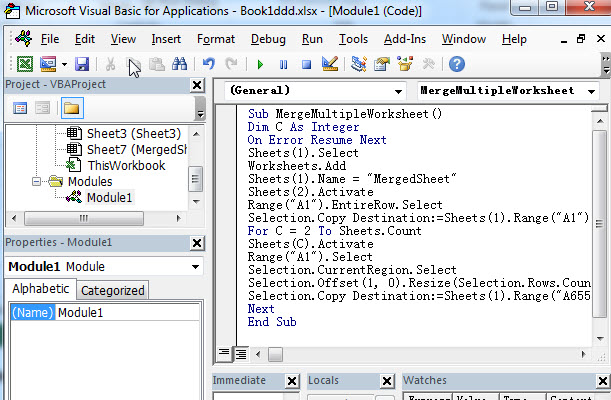5# back to the current worksheet, then run the above excel macro, you will see that a new worksheet named as “MergedSheet” is created and all of worksheets (sheet1, sheet2, sheet3) in current active workbook are combined into the newly worksheet.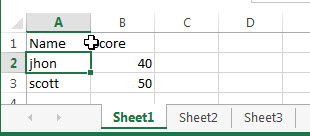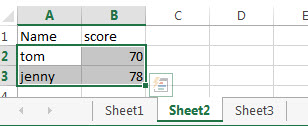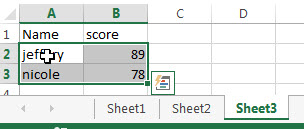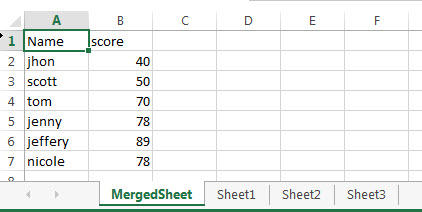You should keep in mind that all of the source worksheets must have the same structure containing same column order and same column headings. Or the VBA code will not work correctly.

### Related Posts

Related Posts
VBA Macro For VLOOKUP From Another Sheet

In the previous post, you should know that how to fix or remove the #N/A error when using VLOOKUP formula to lookup value from another sheet. And this post will show you how to use VBA code to vlookup data ...

How To Insert Comments in Protected Worksheet in Excel

This post will show you how to allow comments in a protected worksheet in Excel. You can easily to insert comments into cells in a normal worksheet in Excel, but if want to insert a comment in a worksheet that ...

How To Convert Text to Upper Cases(Using VBA) in Excel

This post will show you how to switch from lower case to upper case in Excel. and I am going to show you two different ways of converting text to upper cases using formula or VBA macro in Excel 2013,Excel ...

How To Hide Every Other Row in Excel (Using VBA)

This post will show you how to hide alternate rows or columns in Excel or how to hide every third, fourth, fifth row or column in Excel. If you want to hide every other row in your current worksheet, how ...

How to Disable the Save As Prompt in Excel

This post will show you how to use a VBA Macro to save an Excel file and overwrite any existing file without a prompt so that you are going to get the little window that says file already exists do ...

How to Count Cells that Contain even or odd numbers in Excel

This post will guide you how to count the number of cells that contain odd or even numbers within a range of cells using a formula in Excel 2013/2016.How do I count cells that contain odd numbers through the use ...

How to Count Cells that Contain negative Numbers in Excel

This post will guide you how to count the number of cells that contain negative numbers within a range of cells using a formula in Excel 2013/2016.You can count the number of negative numbers in your data using easy functions ...

How to Count Cells Are Not Blank or Empty in Excel

This post will guide you how to count cells that are not blank or empty in a given range cells using a formula in Excel 2013/2016.How do I count the number of cells that are not blank in a particular ...

How to Count Cells Not equal to X or Y in Excel

This post will guide you how to count the number of cells not equal to criteria X or Y in a given range cells using a formula in Excel 2013/2016.You can easily to count cells equal to or not equal ...

How to Count Cells Less Than a Specific Value in Excel

This post will guide you how to count the number of cells less than a particular numeric value in a given range cells using a formula in Excel 2013/2016. How do I count cells that are less than a specific ...

Sidebar